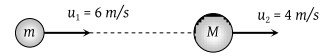A cannon ball is fired with a velocity 200 m/sec at an angle of 60° with the horizontal. At the highest point of its flight ,it explodes into 3 equal fragments, one going vertically upwards with a velocity 100 m/sec, the second one falling vertically downwards with a velocity 100 m/sec. The third fragment will be moving with a velocity

(1) 100 m/s in the horizontal direction

(2) 300 m/s in the horizontal direction

(3) 300 m/s in a direction making an angle of 60° with the horizontal

(4) 200 m/s in a direction making an angle of 60° with the horizontal

Concept Questions :-

Linear momentum
High Yielding Test Series + Question Bank - NEET 2020

Difficulty Level:

A body of mass 5 kg explodes at rest into three fragments with masses in the ratio 1 : 1 : 3. The fragments with equal masses fly in mutually perpendicular directions with speeds of 21 m/s. The velocity of the heaviest fragment will be

(1) 11.5 m/s

(2) 14.0 m/s

(3) 7.0 m/s

(4) 9.89 m/s

Concept Questions :-

Linear momentum
High Yielding Test Series + Question Bank - NEET 2020

Difficulty Level:

A 238U nucleus decays by emitting an alpha particle of speed v ms–1. The recoil speed of the residual nucleus is (in ms–1

(1) –4v/234

(2) v/4

(3) –4v/238

(4) 4v/238

Concept Questions :-

Linear momentum
High Yielding Test Series + Question Bank - NEET 2020

Difficulty Level:

A smooth sphere of mass M moving with velocity u directly collides elastically with another sphere of mass m at rest. After collision their final velocities are V and v respectively. The value of v is

(1) $\frac{2uM}{m}$

(2) $\frac{2um}{M}$

(3) $\frac{2u}{1+\frac{m}{M}}$

(4) $\frac{2u}{1+\frac{M}{m}}$

Concept Questions :-

Linear momentum
High Yielding Test Series + Question Bank - NEET 2020

Difficulty Level:

A particle of mass m moving with horizontal speed 6 m/sec as shown in figure. If m << M then for one dimensional elastic collision, the speed of lighter particle after collision will be(1) 2m/sec in original direction

(2) 2 m/sec opposite to the original direction

(3) 4 m/sec opposite to the original direction

(4) 4 m/sec in original direction

Concept Questions :-

Linear momentum
High Yielding Test Series + Question Bank - NEET 2020

Difficulty Level:

A shell of mass m moving with velocity v suddenly breaks into 2 pieces. The part having mass m/4 remains stationary. The velocity of the other shell will be

(1) v

(2) 2v

(3) $\frac{3}{4}v$

(4) $\frac{4}{3}v$

Concept Questions :-

Linear momentum
High Yielding Test Series + Question Bank - NEET 2020

Difficulty Level:

Two equal masses m1 and m2 moving along the same straight line with velocities +3 m/s and –5 m/s respectively collide elastically. Their velocities after the collision will be respectively

(1) +4 m/s for both

(2) –3 m/s and +5 m/s

(3) –4 m/s and +4 m/s

(4) –5 m/s and +3 m/s

Concept Questions :-

Linear momentum
High Yielding Test Series + Question Bank - NEET 2020

Difficulty Level:

A body falls on a surface of coefficient of restitution 0.6 from a height of 1 m. Then the body rebounds to a height of

(1) 0.6 m

(2) 0.4 m

(3) 1 m

(4) 0.36 m

Concept Questions :-

Linear momentum
High Yielding Test Series + Question Bank - NEET 2020

Difficulty Level:

A body at rest breaks up into 3 parts. If 2 parts having equal masses fly off perpendicularly each after with a velocity of 12m/s, then the velocity of the third part which has 3 times mass of each part is

(1) $4\sqrt{2}\text{\hspace{0.17em}}m/s$ at an angle of 45° from each body

(2) $24\sqrt{2}\text{\hspace{0.17em}}m/s$ at an angle of 135° from each body

(3) $6\sqrt{2}\text{\hspace{0.17em}}m/s$ at 135° from each body

(4) $4\sqrt{2}\text{\hspace{0.17em}}m/s$ at 135° from each body

Concept Questions :-

Linear momentum
High Yielding Test Series + Question Bank - NEET 2020

Difficulty Level:

A particle falls from a height h upon a fixed horizontal plane and rebounds. If e is the coefficient of restitution, the total distance travelled before rebounding has stopped is

(1) $h\left(\frac{1+{e}^{2}}{1-{e}^{2}}\right)$

(2) $h\left(\frac{1-{e}^{2}}{1+{e}^{2}}\right)$

(3) $\frac{h}{2}\left(\frac{1-{e}^{2}}{1+{e}^{2}}\right)$

(4) $\frac{h}{2}\left(\frac{1+{e}^{2}}{1-{e}^{2}}\right)$

Concept Questions :-

Linear momentum